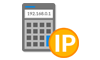## Determining Subnets Available

##### How to determine the number of subnets possible:
If we want to determine the numbers of subnets possible given a classful or CIDR notation, we count the number of ones in the host octet(s) of the subnet mask.

If we are provided the network address written in CIDR as 192.168.5.0/20, we would write out the prefix in binary form as below, where “n” is a network bit and “h” is a host bit.

```                    1 1 1 1 1 1 1 1 . 1 1 1 1 1 1 1 1 . 1 1 1 1 0 0 0 0 . 0 0 0 0 0 0 0 0
n n n n n n n n . n n n n n n n n . n n n n h h h h . h h h h h h h h
```

To figure out the number of subnets, we count the number of network bits in the octet responsible for subnetting. In our example above, we are looking at the third octet and we count the number of ones for the network bits. We have 4 ones in the subnet mask for the host bits.

If you are using non-CIDR/classful subnetting, this follows RFC 950 where it was recommended to reserve the subnet values consisting of all zeros and all ones. This reduced the number of available subnets by two.

The formula to use if we are following RFC 950 is below, where n = the number of network bits:

(2n) – 2

In our example, this would be:

(24) – 2 = (16 – 2) = 14 usable subnets in a /20 subnet.

However due to the inefficiencies with RFC 950, it is typically abandoned for use on the Internet. Most modern networking gear follows RFC 1878 which supports networks utilizing all 0’s and all 1’s in the subnet mask.

The formula to use if we are following RFC 1878 is below, where n = the number of network bits:

2n

In our example, this would be:

(24) = 16 usable subnets in a /20 CIDR notation.